Subject:
Ratios and Proportions
Material Type:
Lesson Plan
Level:
Middle School
7
Provider:
Pearson
Tags:
• Percentages
Language:
English
Media Formats:
Text/HTML

# Percent Increase Problems## Overview

Students represent and solve percent increase problems.

# Key Concepts

When there is a percent increase between a starting amount and a final amount, the relationship can be represented by an equation of the form y = kx where y is the final amount, x is the starting amount, and k is the constant of proportionality, which is equal to 1 plus the percent change, p, represented as a decimal: k = 1 + p, so y = (1 + p)x.

The constant of proportionality k has the value it does—a number greater than 1—because of the way the distributive property can be used to simplify the expression for the starting amount increased by a percent of the starting amount: x + x(p) = x(1 + p).

# Goals and Learning Objectives

• Determine the unknown amount—either the starting amount, the percent change, or the final amount—in a percent increase situation when given the other two amounts.
• Make a table to represent a percent increase problem.
• Write and solve an equation to represent a percent increase problem.

# Lesson Guide

• Have students read the prompt and the question.
• Have partners share their ideas about other situations that might involve percent increases.

SWD: Whenever providing these prompts and questions to students with disabilities, make sure they understand the meaning of included domain-specific vocabulary. Review and reinforce the meaning of domain-specific terms to promote comprehension and recall.

# Mathematics

This lesson sets up thinking about proportional relationships in a different way from how they have been presented so far in the unit. Problems involving percent increase and percent decrease can be confusing for many students, but linking the idea of percent change to the structure of proportional relationships provides students with resources for thinking about these concepts that can help clarify what is going on.

# Percent Increase Problems

The sales tax problems you just worked on are examples of percent increase problems. Discuss the following with your partner.

• Can you think of other situations that involve percent increase?

# Lesson Guide

Discuss the Math Mission. Students will represent and solve percent increase problems.

SWD: If students seem unsure of the task, model the steps for finding the percent increase with them before asking them to do the problems themselves.

## Opening

Represent and solve percent increase problems.

# Lesson Guide

Have students work in pairs on all problems and the presentation.

# Mathematical Practices

Mathematical Practice 7: Look for and make use of structure.

Students use the structure of the relationships among quantities to reason about solutions that make sense.

Mathematical Practice 2: Reason abstractly and quantitatively.

Students use the situations described to reason about whether solutions make sense given their meaning in the situation and given the values in the situation.

# Interventions

[common error] Student sets up an incorrect equation.

• Is the unknown amount the starting amount, final amount, or percent change? Where is the unknown amount in your equation?
• Does your solution make sense in this situation? If not, check the structure of your equation. Does it make sense?

[common error] Student sets up a correct equation, but solves it incorrectly.

• Does your solution make sense in this situation?
• Should your solution be greater than or less than the final amount [starting amount]?

Student completes problems efficiently and correctly.

• Create a new problem based on one of the problems you just solved that involves a percent decrease rather than a percent increase. Is the structure of the equation the same or different? Explain.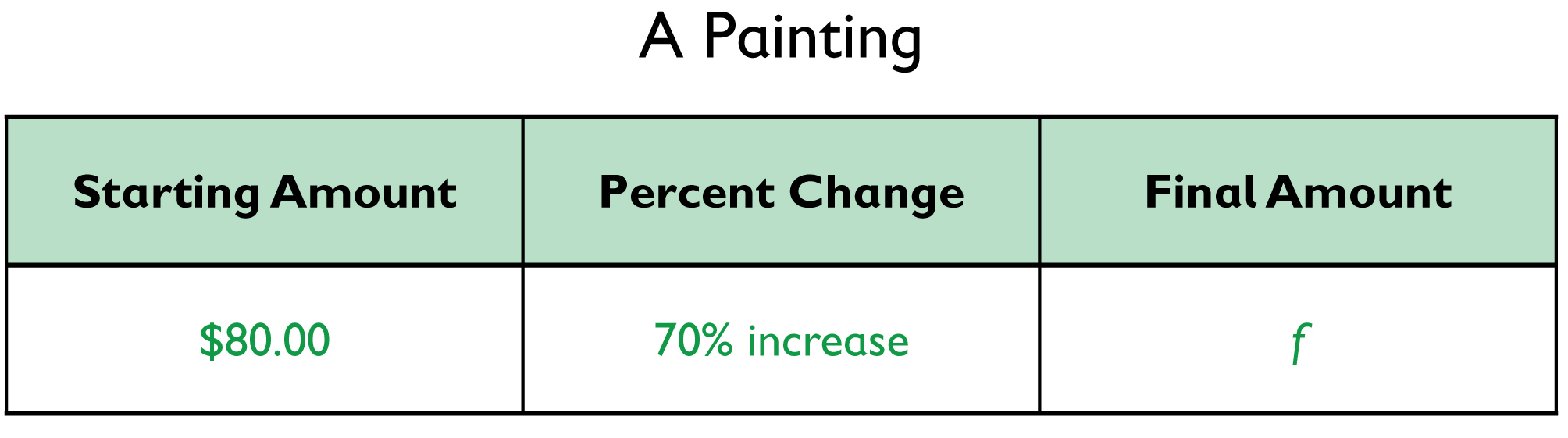• Equation:

f = 80 + 0.7(80)

= (1 + 0.7)80

= (1.7)80

• Solution: f = (1.7)80f = 136
The painting is now worth $136.00. ## Work Time # A Painting Three years ago, Mr. Okawa bought a painting for$80. Since then, the value of the painting has increased by 70%. What is the painting worth now?

• Copy and complete the table.
• Write an equation.
• Write the solution as a complete sentence.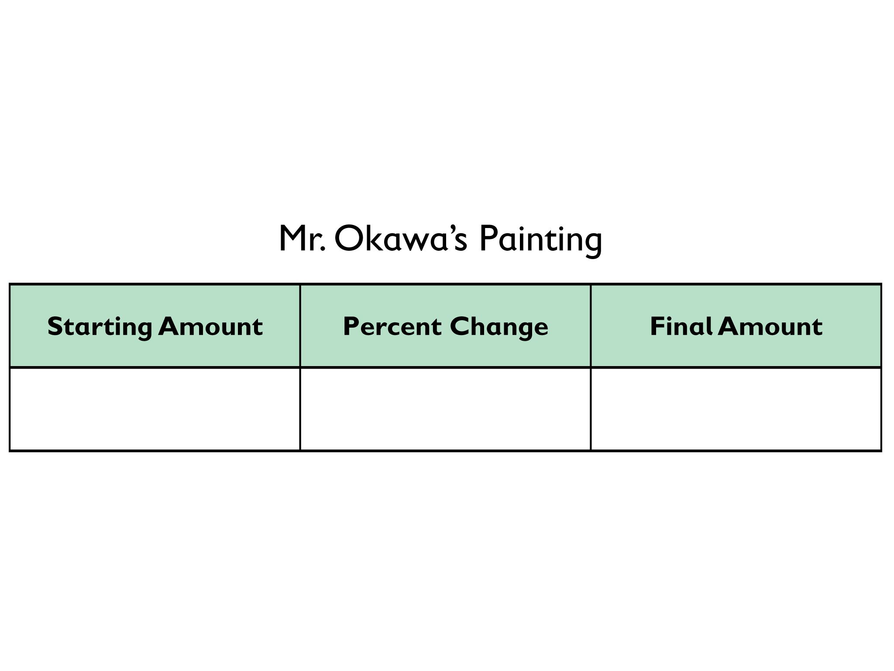## Hint:

What do you know? What do you need to find out?

# Lesson Guide

Have students work in pairs on all problems and the presentation.

# Interventions

[common error] Student sets up an incorrect equation.

• Is the unknown amount the starting amount, final amount, or percent change? Where is the unknown amount in your equation?
• Does your solution make sense in this situation? If not, check the structure of your equation. Does it make sense?

[common error] Student sets up a correct equation, but solves it incorrectly.

• Does your solution make sense in this situation?
• Should your solution be greater than or less than the final amount [starting amount]?

Student completes problems efficiently and correctly.

• Create a new problem based on one of the problems you just solved that involves a percent decrease rather than a percent increase. Is the structure of the equation the same or different? Explain.

• Equation:

25.20 = s + 0.05s

= (1 + 0.05)s

= (1.05)s

• Solution:

25.20 = (1.05)s

$\frac{25.20}{1.05}=\frac{\left(1.05\right)s}{1.05}$

24 = s

Angela was earning $24.00 per hour before the pay increase. ## Work Time # Hourly Wage A company increases the wages of all its employees by 5%. Angela now earns$25.20 per hour. What was she earning before the pay increase?

• Copy and complete the table.
• Write an equation.
• Write the solution as a complete sentence.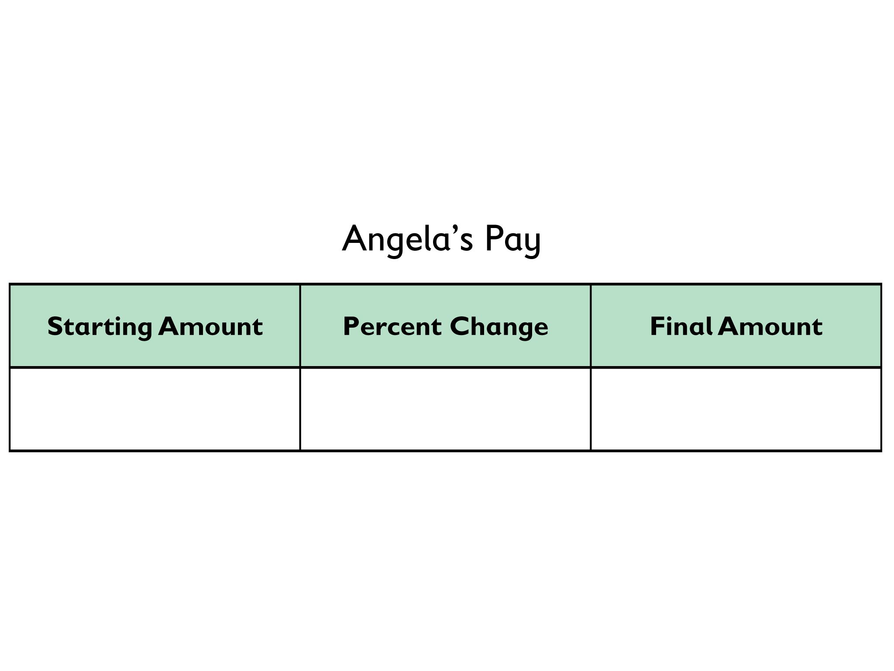## Hint:

What do you know? What do you need to find out?

# Lesson Guide

Have students work in pairs on all problems and the presentation.

# Interventions

[common error] Student sets up an incorrect equation.

• Does your solution make sense in this situation? If not, check the structure of your equation. Does it make sense?
• What should be multiplied by the percent change in your equation for Jon’s problem?

[common error] Student sets up a correct equation, but solves it incorrectly.

• Does your solution make sense in this situation?
• For Jon’s problem, should your solution be greater than or less than 100%?
• For Jon’s problem, look at the starting amount and final amount, and make an estimate of the percent increase between them. Is your solution close to your estimate?

Student completes problems efficiently and correctly.

• Create a new problem based on one of the problems you just solved that involves a percent decrease rather than a percent increase. Is the structure of the equation the same or different? Explain.

•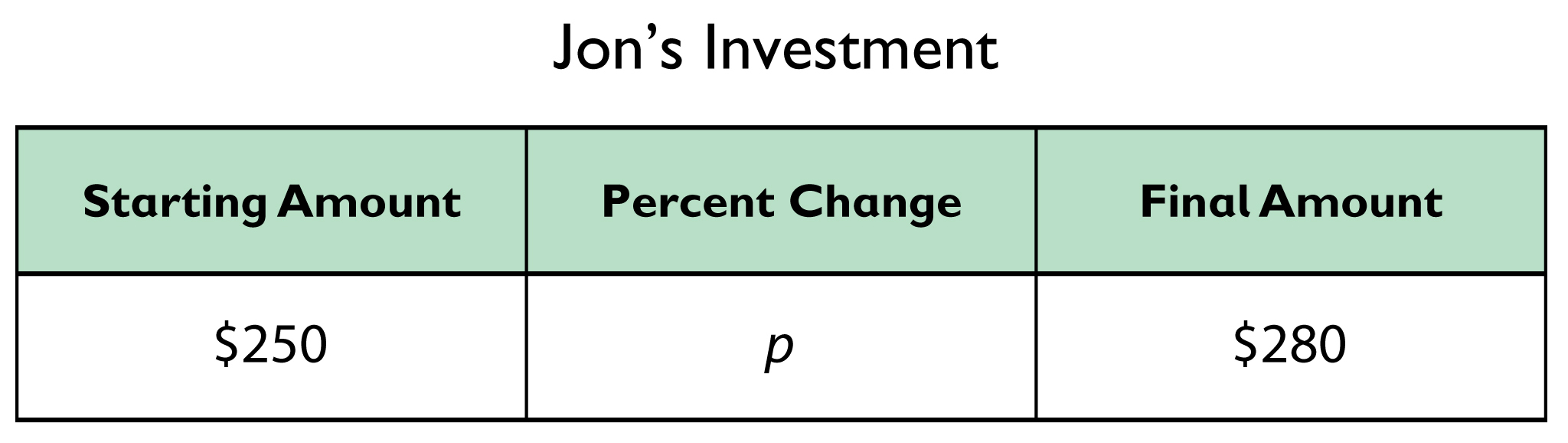• 280 = 250 + 250p

280 = 250(1 + p)

Equation: $p=\frac{280}{250}-1$

• Solution: $p=\frac{280}{250}-1$

p = 0.12

The value of Jon’s investment increased by 12%.

# Jon’s Investment

Jon invested $250 for one year. He now has$280. What is the percent increase?

• Copy and complete the table.
• Write an equation.
• Write the solution as a complete sentence.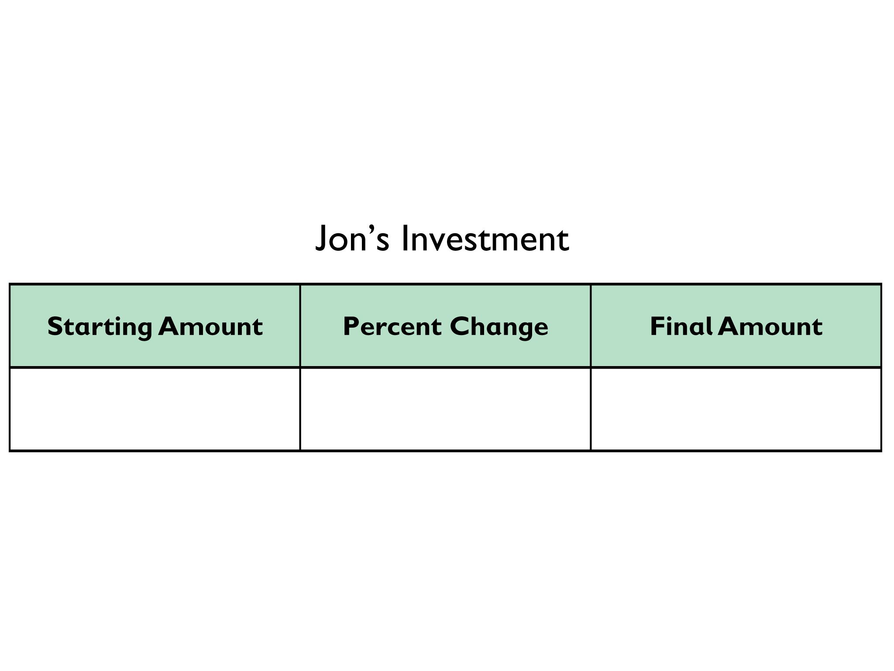## Hint:

What do you know? What do you need to find out?

# Preparing for Ways of Thinking

Look and listen for students who:

• Understand that each relationship between starting and final amounts is a proportional relationship.
• Understand why each constant of proportionality has the value it does.
• Make explicit use of the distributive property to write or simplify equations in each problem.

# Challenge Problem

• Answers will vary. Ideally, students will demonstrate an understanding of the fact that an amount, x, that is increased by 100%, equals the amount plus the amount multiplied by 1.00: x + 1(x). In other words, x(1 + 1) = 2x, which is double the amount.

# Prepare a Presentation

Prepare a presentation in which you show and explain each part of your work for all three of the percent increase problems.

# Challenge Problem

• Explain why saying that an amount has doubled is the same as saying that an amount has increased by 100%.

# Mathematics

Highlight student work that includes explicit application of the distributive property in writing, simplifying, and solving the equations. Then ask for students to present work that helps bring out why the constant of proportionality has the value it does in each case and why the relationships between starting and final amounts are proportional relationships in each case. Finally, highlight the mathematical practices at work in student thinking— Mathematical Practice 7: Look for and make use of structure, and Mathematical Practice 2: Reason abstractly and quantitatively.

# Ways of Thinking: Make Connections

Take notes about your classmates’ approaches and explanations for solving the three percent increase problems.

## Hint:

• Is the unknown amount the starting amount, the final amount, or the percent change?
• Where is the unknown amount in your equation?
• Why does your solution make sense in this situation?
• Should the solution be greater than or less than the given amount?
• Should the solution be greater than or less than 100%?
• How did you use the mathematical structure of the situation to help you solve the problem?

# A Possible Summary

When there is a percent increase between a starting amount and a final amount, the relationship can be represented by an equation of the form y = kx where y is the final amount, x is the starting amount, and k is the constant of proportionality, which is equal to 1 plus the percent change, represented as a decimal. The constant of proportionality k has the value it does—a number greater than 1—because of the way the distributive property can be used to simplify the expression for the starting amount increased by a percent of the starting amount.

ELL: When discussing percent increase as related to the constant of proportionality, make a point of writing the Additional Discussion Points on the board, along with students’ responses. This will assist ELLs by giving them written and oral access to the questions. Have students write important information in their Notebook.

Discuss the following concepts:

• There are many different kinds of situations involving percent increase.
• No matter what the context, a table can help you organize the information so you can solve the problem.

# Summary of the Math: Percent Increase

Write a summary about representing and solving problems that involve percent increase.

## Hint:

• Do you explain the relationship between the starting amount, percent increase, and final amount?
• Do you explain how to write an equation that you can use to solve a percent increase problem?
• Do you describe how to use a table to organize the information in a percent increase problem?
• Do you give examples of situations that involve percent increase?

# Lesson Guide

Have each student write a brief reflection before the end of the class. Review the reflections to find out what students identified as being the same about the three problems they solved.

ELL: Provide ELLs access to a dictionary and make sure they are given time to discuss with a partner before writing, to help them organize their thoughts. Allow ELLs who share the same primary language to discuss in their language of choice.

# Reflection

Write a reflection about the ideas discussed in class today. Use the sentence starter below if you find it to be helpful.

One thing that is the same about the three problems I solved today is …